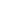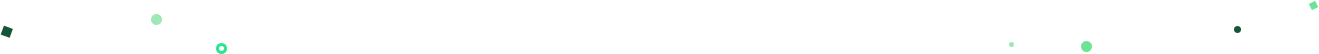## Mode Studio

The Collaborative Data Science Platform# SQL Window Functions

Starting here? This lesson is part of a full-length tutorial in using SQL for Data Analysis. Check out the beginning.

In this lesson we'll cover:

This lesson uses data from Washington DC's Capital Bikeshare Program, which publishes detailed trip-level historical data on their website. The data was downloaded in February, 2014, but is limited to data collected during the first quarter of 2012. Each row represents one ride. Most fields are self-explanatory, except `rider_type`: "Registered" indicates a monthly membership to the rideshare program, "Casual" incidates that the rider bought a 3-day pass. The `start_time` and `end_time` fields were cleaned up from their original forms to suit SQL date formatting—they are stored in this table as timestamps.

## Intro to window functions

PostgreSQL's documentation does an excellent job of introducing the concept of Window Functions:

A window function performs a calculation across a set of table rows that are somehow related to the current row. This is comparable to the type of calculation that can be done with an aggregate function. But unlike regular aggregate functions, use of a window function does not cause rows to become grouped into a single output row — the rows retain their separate identities. Behind the scenes, the window function is able to access more than just the current row of the query result.

The most practical example of this is a running total:

``````SELECT duration_seconds,
SUM(duration_seconds) OVER (ORDER BY start_time) AS running_total
FROM tutorial.dc_bikeshare_q1_2012
``````

You can see that the above query creates an aggregation (`running_total`) without using `GROUP BY`. Let's break down the syntax and see how it works.

## Basic windowing syntax

The first part of the above aggregation, `SUM(duration_seconds)`, looks a lot like any other aggregation. Adding `OVER` designates it as a window function. You could read the above aggregation as "take the sum of `duration_seconds` over the entire result set, in order by `start_time`."

If you'd like to narrow the window from the entire dataset to individual groups within the dataset, you can use `PARTITION BY` to do so:

``````SELECT start_terminal,
duration_seconds,
SUM(duration_seconds) OVER
(PARTITION BY start_terminal ORDER BY start_time)
AS running_total
FROM tutorial.dc_bikeshare_q1_2012
WHERE start_time < '2012-01-08'
``````

The above query groups and orders the query by `start_terminal`. Within each value of `start_terminal`, it is ordered by `start_time`, and the running total sums across the current row and all previous rows of `duration_seconds`. Scroll down until the `start_terminal` value changes and you will notice that `running_total` starts over. That's what happens when you group using `PARTITION BY`. In case you're still stumped by `ORDER BY`, it simply orders by the designated column(s) the same way the `ORDER BY` clause would, except that it treats every partition as separate. It also creates the running total—without `ORDER BY`, each value will simply be a sum of all the `duration_seconds` values in its respective `start_terminal`. Try running the above query without `ORDER BY` to get an idea:

``````SELECT start_terminal,
duration_seconds,
SUM(duration_seconds) OVER
(PARTITION BY start_terminal) AS start_terminal_total
FROM tutorial.dc_bikeshare_q1_2012
WHERE start_time < '2012-01-08'
``````

The `ORDER` and `PARTITION` define what is referred to as the "window"—the ordered subset of data over which calculations are made.

Note: You can't use window functions and standard aggregations in the same query. More specifically, you can't include window functions in a `GROUP BY` clause.

## Practice Problem

Write a query modification of the above example query that shows the duration of each ride as a percentage of the total time accrued by riders from each start_terminal

Try it out See the answer

## The usual suspects: SUM, COUNT, and AVG

When using window functions, you can apply the same aggregates that you would under normal circumstances—`SUM`, `COUNT`, and `AVG`. The easiest way to understand these is to re-run the previous example with some additional functions. Make

``````SELECT start_terminal,
duration_seconds,
SUM(duration_seconds) OVER
(PARTITION BY start_terminal) AS running_total,
COUNT(duration_seconds) OVER
(PARTITION BY start_terminal) AS running_count,
AVG(duration_seconds) OVER
(PARTITION BY start_terminal) AS running_avg
FROM tutorial.dc_bikeshare_q1_2012
WHERE start_time < '2012-01-08'
``````

Alternatively, the same functions with `ORDER BY`:

``````SELECT start_terminal,
duration_seconds,
SUM(duration_seconds) OVER
(PARTITION BY start_terminal ORDER BY start_time)
AS running_total,
COUNT(duration_seconds) OVER
(PARTITION BY start_terminal ORDER BY start_time)
AS running_count,
AVG(duration_seconds) OVER
(PARTITION BY start_terminal ORDER BY start_time)
AS running_avg
FROM tutorial.dc_bikeshare_q1_2012
WHERE start_time < '2012-01-08'
``````

Make sure you plug those previous two queries into Mode and run them. This next practice problem is very similar to the examples, so try modifying the above code rather than starting from scratch.

## Practice Problem

Write a query that shows a running total of the duration of bike rides (similar to the last example), but grouped by `end_terminal`, and with ride duration sorted in descending order.

Try it out See the answer

## ROW_NUMBER()

`ROW_NUMBER()` does just what it sounds like—displays the number of a given row. It starts are 1 and numbers the rows according to the `ORDER BY` part of the window statement. `ROW_NUMBER()` does not require you to specify a variable within the parentheses:

``````SELECT start_terminal,
start_time,
duration_seconds,
ROW_NUMBER() OVER (ORDER BY start_time)
AS row_number
FROM tutorial.dc_bikeshare_q1_2012
WHERE start_time < '2012-01-08'
``````

Using the `PARTITION BY` clause will allow you to begin counting 1 again in each partition. The following query starts the count over again for each terminal:

``````SELECT start_terminal,
start_time,
duration_seconds,
ROW_NUMBER() OVER (PARTITION BY start_terminal
ORDER BY start_time)
AS row_number
FROM tutorial.dc_bikeshare_q1_2012
WHERE start_time < '2012-01-08'
``````

## RANK() and DENSE_RANK()

`RANK()` is slightly different from `ROW_NUMBER()`. If you order by `start_time`, for example, it might be the case that some terminals have rides with two identical start times. In this case, they are given the same rank, whereas `ROW_NUMBER()` gives them different numbers. In the following query, you notice the 4th and 5th observations for `start_terminal` 31000—they are both given a rank of 4, and the following result receives a rank of 6:

``````SELECT start_terminal,
duration_seconds,
RANK() OVER (PARTITION BY start_terminal
ORDER BY start_time)
AS rank
FROM tutorial.dc_bikeshare_q1_2012
WHERE start_time < '2012-01-08'
``````

You can also use `DENSE_RANK()` instead of `RANK()` depending on your application. Imagine a situation in which three entries have the same value. Using either command, they will all get the same rank. For the sake of this example, let's say it's "2." Here's how the two commands would evaluate the next results differently:

• `RANK()` would give the identical rows a rank of 2, then skip ranks 3 and 4, so the next result would be 5
• `DENSE_RANK()` would still give all the identical rows a rank of 2, but the following row would be 3—no ranks would be skipped.

## Practice Problem

Write a query that shows the 5 longest rides from each starting terminal, ordered by terminal, and longest to shortest rides within each terminal. Limit to rides that occurred before Jan. 8, 2012.

Try it out See the answer

## NTILE

You can use window functions to identify what percentile (or quartile, or any other subdivision) a given row falls into. The syntax is `NTILE(*# of buckets*)`. In this case, `ORDER BY` determines which column to use to determine the quartiles (or whatever number of 'tiles you specify). For example:

``````SELECT start_terminal,
duration_seconds,
NTILE(4) OVER
(PARTITION BY start_terminal ORDER BY duration_seconds)
AS quartile,
NTILE(5) OVER
(PARTITION BY start_terminal ORDER BY duration_seconds)
AS quintile,
NTILE(100) OVER
(PARTITION BY start_terminal ORDER BY duration_seconds)
AS percentile
FROM tutorial.dc_bikeshare_q1_2012
WHERE start_time < '2012-01-08'
ORDER BY start_terminal, duration_seconds
``````

Looking at the results from the query above, you can see that the `percentile` column doesn't calculate exactly as you might expect. If you only had two records and you were measuring percentiles, you'd expect one record to define the 1st percentile, and the other record to define the 100th percentile. Using the `NTILE` function, what you'd actually see is one record in the 1st percentile, and one in the 2nd percentile. You can see this in the results for `start_terminal` 31000—the `percentile` column just looks like a numerical ranking. If you scroll down to `start_terminal` 31007, you can see that it properly calculates percentiles because there are more than 100 records for that `start_terminal`. If you're working with very small windows, keep this in mind and consider using quartiles or similarly small bands.

## Practice Problem

Write a query that shows only the duration of the trip and the percentile into which that duration falls (across the entire dataset—not partitioned by terminal).

Try it out See the answer

It can often be useful to compare rows to preceding or following rows, especially if you've got the data in an order that makes sense. You can use `LAG` or `LEAD` to create columns that pull values from other rows—all you need to do is enter which column to pull from and how many rows away you'd like to do the pull. `LAG` pulls from previous rows and `LEAD` pulls from following rows:

``````SELECT start_terminal,
duration_seconds,
LAG(duration_seconds, 1) OVER
(PARTITION BY start_terminal ORDER BY duration_seconds) AS lag,
(PARTITION BY start_terminal ORDER BY duration_seconds) AS lead
FROM tutorial.dc_bikeshare_q1_2012
WHERE start_time < '2012-01-08'
ORDER BY start_terminal, duration_seconds
``````

This is especially useful if you want to calculate differences between rows:

``````SELECT start_terminal,
duration_seconds,
duration_seconds -LAG(duration_seconds, 1) OVER
(PARTITION BY start_terminal ORDER BY duration_seconds)
AS difference
FROM tutorial.dc_bikeshare_q1_2012
WHERE start_time < '2012-01-08'
ORDER BY start_terminal, duration_seconds
``````

The first row of the `difference` column is null because there is no previous row from which to pull. Similarly, using `LEAD` will create nulls at the end of the dataset. If you'd like to make the results a bit cleaner, you can wrap it in an outer query to remove nulls:

``````SELECT *
FROM (
SELECT start_terminal,
duration_seconds,
duration_seconds -LAG(duration_seconds, 1) OVER
(PARTITION BY start_terminal ORDER BY duration_seconds)
AS difference
FROM tutorial.dc_bikeshare_q1_2012
WHERE start_time < '2012-01-08'
ORDER BY start_terminal, duration_seconds
) sub
WHERE sub.difference IS NOT NULL
``````

## Defining a window alias

If you're planning to write several window functions in to the same query, using the same window, you can create an alias. Take the `NTILE` example above:

``````SELECT start_terminal,
duration_seconds,
NTILE(4) OVER
(PARTITION BY start_terminal ORDER BY duration_seconds)
AS quartile,
NTILE(5) OVER
(PARTITION BY start_terminal ORDER BY duration_seconds)
AS quintile,
NTILE(100) OVER
(PARTITION BY start_terminal ORDER BY duration_seconds)
AS percentile
FROM tutorial.dc_bikeshare_q1_2012
WHERE start_time < '2012-01-08'
ORDER BY start_terminal, duration_seconds
``````

This can be rewritten as:

``````SELECT start_terminal,
duration_seconds,
NTILE(4) OVER ntile_window AS quartile,
NTILE(5) OVER ntile_window AS quintile,
NTILE(100) OVER ntile_window AS percentile
FROM tutorial.dc_bikeshare_q1_2012
WHERE start_time < '2012-01-08'
WINDOW ntile_window AS
(PARTITION BY start_terminal ORDER BY duration_seconds)
ORDER BY start_terminal, duration_seconds
``````

The `WINDOW` clause, if included, should always come after the `WHERE` clause.